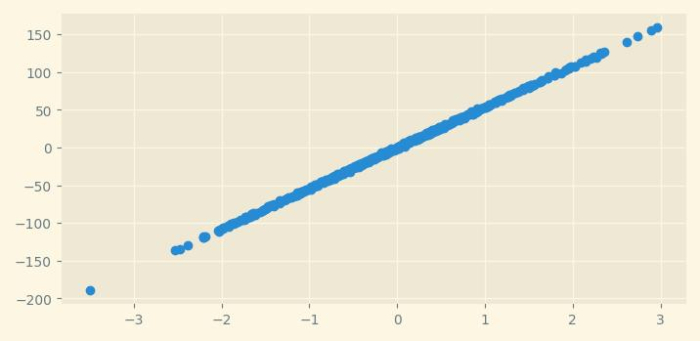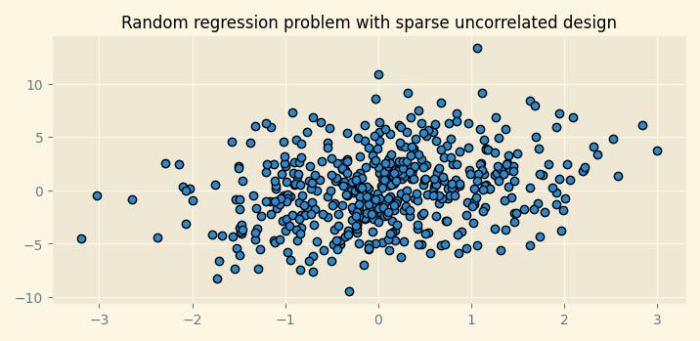# How to generate random regression problems using Python Scikit-learn?

Python Scikit-learn provides us make_regression() function with the help of which we can generate a random regression problem. In this tutorial, we will learn to generate random regression problems and random regression problems with sparse uncorrelated design.

## Random Regression Problem

To generate a random regression problem using Python Scikit-learn, we can follow the below given steps −

Step 1 − Import the libraries sklearn.datasets.make_regression and matplotlib which are necessary to execute the program.

Step 2 − Provide the number of samples and other parameters.

Step 3 − Use matplotlib library to set the size and style of the output figure.

Step 4 − Plot the regression problem using matplotlib.

### Example

In the below example, we will be generating regression problem with 500 samples.

# Importing libraries
from sklearn.datasets import make_regression
from matplotlib import pyplot as plt
from matplotlib import style
import seaborn as sns

# Set the figure size
plt.rcParams["figure.figsize"] = [7.50, 3.50]
plt.rcParams["figure.autolayout"] = True

# Creating and plotting the regression problem
style.use("Solarize_Light2")

r_data, r_values = make_regression(n_samples=500, n_features=1, n_informative=2, noise=1)

plt.scatter(r_data[:,0],r_values,cmap='rocket');
plt.show()


### Output

It will produce the following output −## Random Regression Problem with Sparse Uncorrelated Design

Python Scikit-learn provides us make_sparse_uncorrelated() function with the help of which we can generate a random regression problem with uncorrelated design.

To do so, we can take the below given steps −

Step 1 − Import the libraries sklearn.datasets.make_sparse_uncorrelated and matplotlib which are necessary to execute the program.

Step 2 − Provide the number of samples and other parameters.

Step 3 − Use matplotlib library to set the size and style of the output figure.

Step 4 − Plot the regression problem using matplotlib.

### Example

In the below example, we will be generating regression problem with 500 samples and 4 features. The by default value of n_features parameter is 10.

# Importing libraries
from sklearn.datasets import make_sparse_uncorrelated
from matplotlib import pyplot as plt
from matplotlib import style

# Set the figure size
plt.rcParams["figure.figsize"] = [7.50, 3.50]
plt.rcParams["figure.autolayout"] = True

# Creating the regression problem with sparse uncorrelated design
X, y = make_sparse_uncorrelated(n_samples=500, n_features=4)

# Plotting the dataset
style.use("Solarize_Light2")
plt.figure(figsize=(7.50, 3.50))
plt.title("Random regression problem with sparse uncorrelated design", fontsize="12")
plt.scatter(X[:,0],y,edgecolor="k");
plt.show()


### Output

It will produce the following output −